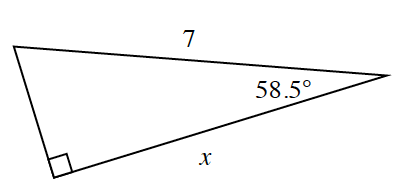### Home > INT2 > Chapter 8 > Lesson 8.4.2 > Problem8-98

8-98.Krista is trying to solve for $x$ in the triangle at right.

1. What equation could Krista write?

With the given angle of $58.5^\circ$, $x$ is the adjacent side and $7$ is the hypotenuse.
Which trigonometry ratio should you use?

2.Krista does not have a scientific calculator with trig buttons on it, but she does remember something funny her friend Juanisha told her. Juanisha’s favorite sine ratio is $\sin31.5^\circ\approx0.522$ because $5/22$ is Juanisha’s birthday! Without using the trig buttons on your calculator, use Juanisha’s favorite ratio to solve your equation from part (a).

Remember the sine of one acute angle in a right triangle equals the cosine of the other acute angle.
Find the measure of the missing angle in the triangle, then use sine ratio.